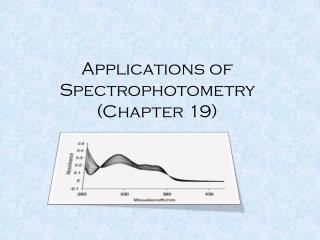# Applications of Spectrophotometry (Chapter 19) - PowerPoint PPT PresentationDownload PresentationApplications of Spectrophotometry (Chapter 19)

Applications of Spectrophotometry (Chapter 19)Download Presentation## Applications of Spectrophotometry (Chapter 19)

- - - - - - - - - - - - - - - - - - - - - - - - - - - E N D - - - - - - - - - - - - - - - - - - - - - - - - - - -
##### Presentation Transcript

1. Applications of Spectrophotometry(Chapter 19)

2. Red shiftof lmax with increasing conjugation CH2=CHCH2CH2CH=CH2 lmax =185 nm CH2=CHCH=CH2lmax =217 nm vs. Red shiftof lmax with # of rings Benzene lmax =204 nm Naphthalene lmax =286 nm

3. Polar solvents more likely to shift absorption maxima Shifts of lmax with solvent polarity n®p* hypsochromic/blue shift p®p* bathochromic/red shift

4. Hypsochromic shift heptane methanol

5. Generally, extending conjugation leads to red shift “particle in a box” QM theory; bigger box Substituents attached to a chromophore that cause a red shift are called “auxochromes” Strain has an effect… max 253 239 256 248

6. Generally, extending conjugation leads to red shift “particle in a box” QM theory; bigger box

7. Absorbance Determination of Concentration – Multicomponent System C B l1 l2 Wavelength (nm) Absorbance C+B l1 l2 Wavelength (nm)

8. Absorbance Determination of Concentration – Multicomponent System C B l1 l2 Wavelength (nm) (1) Measure eB and eC at l1 and l2 (pure substances)

9. Determination of Concentration – Multicomponent System (2) Measure Aat l1 and l2 (mixture) Absorbance C+B l1 l2 Wavelength (nm)

10. A1 = xeB1 + yeC1 A2 = xeB2 + yeC2 x = molarity B y = molarity C Determination of Concentration – Multicomponent System Absorbance C+B l1 l2 Wavelength (nm)

11. Isobestic Points HIn (acid form) In- (base form) Brocresol green When one absorbing species is converted to another, it is apparent in the absorption spectrum.

12. Isobestic Points pH pH [H+] [OH-] I see an isobestic point!!!

13. Isobestic Points HIn (acid form) In- (base form) The Total concentration of Bromocresol Green is constant throughout the reaction [Hin] + [In-] = F bromocresol green Cx + Cy = C A = b (exCx + eyCy) But at the isosbestic point both molar absorptivities are the same! ex + ey = e

14. Isobestic Points HIn (acid form) In- (base form) ex + ey = e Therefore, the absorbancedoes not depend on the extent of reaction (i.e. on the particular concentrations of x and y) A = b (exCx + eyCy) = b e (Cx+ Cy) = b e C An isobestic point is good evidence that only two principal species are present in a reaction.

15. Isobestic Points - Application Oximetry

16. O2 Hb HbO2 deoxyhemoglobin Oxyhemoglobin 100 % O2 0 % O2

17. Measuring the equilibrium constant (The Scatchard Plot) Biochemistry example: The cellular action of a hormone begins when the hormone (L) Binds to it’s receptor protein (R) in a tight and specific way. The binding thus gives rise to conformational changes which change the biological activity of the receptor (an enzyme, an enzyme regulator, an ion channel, or a regulator of gene expression. R + L RL The binding depends of the concentration of the concentration of the components. Ka is the association constant and Kd is the dissociation constant

18. Measuring the equilibrium constant (The Scatchard Plot) When binding has reached equilibrium, the total number of binding sites, Bmax= [R] + [RL] whereas, the number of unbound sites would be [R] = Bmax– [RL]. The equilibrium constant would then be This expression can then be rearranged to find the ratio of bound to unbound (free) hormone

19. Measuring the equilibrium constant (The Scatchard Plot)

20. The method of continuous variation (for isomolar solution)(Job’s method) A + n B ABn Call this C Absorbance Allows for the determination of the stoichiometry of the Predominant product. 1 0 Mole fraction (c = nb / na+nb)

21. The method of continuous variation (for isomolar solution)(Job’s method) Requirements: The system must conform to Beer's law. Only single equilibrium Equimolar solutions MA = MB Constant volume 1 = VT = VA + VB K reasonably greater than 1 pH and ionic strength must be maintained constant

22. The method of continuous variation (for isomolar solution)(Job’s method) A + n B C M(1-x) Mx 0 Start Change -C -nC +C Equil. M(1-x)-C Mx-nC C M(1-x) is a concentration

23. Corrected Absorbance A3X AX AX2 0 1 0.25 0.5 0.66 Mole fraction (c = nb / na)